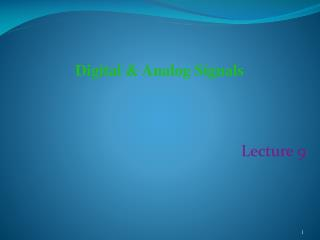DownloadDownload PresentationLecture 9

# Lecture 9

Download Presentation## Lecture 9

- - - - - - - - - - - - - - - - - - - - - - - - - - - E N D - - - - - - - - - - - - - - - - - - - - - - - - - - -
##### Presentation Transcript

1. Digital & Analog Signals Lecture 9

2. Data vs. Signals • Data • Data is what we want to transmit or store • Data is usually stored • Example- Computer Files, Movie on a DVD • Signal • Signals are what we use to transmit the data • Signals are transient (Not permanent) • Example- Telephone Conversation over a telephone Line, Live TV News It is important to understand the difference between data and signal

3. Types of Signals • There are two types of signal • Analog • Digital

4. Analog Signal • Analog signal is a continuous waveform. • examples such as music and video.

5. Digital Signal Digital is a discrete or non-continuous waveform with examples such as computer 1s and 0s.

6. Noise in the Analog Signal A bad signal has noise (disturbance) in it. It is harder to separate noise from an analog signal. Following figure shows analog signal with noise

7. Noise in the Digital Signal Following figure shows noise in the digital signal, but you can still separate a high Voltage from a low voltage. That is why- it is easier to separate noise from the digital signal

8. Too Much Noise If the noise is too much you probably cannot separate signal from the noise- you cannot separate a high voltage from a low voltage.

9. Basic Terms used with signals • Amplitude • Frequency • Phase

10. Amplitude The amplitude of a signal is the height of the wave above or below a given reference point.

11. Frequency • The frequency is the number of times a signal makes a complete cycle within a given time frame. Measured in Hz (hertz = cycles/second) Period is the length of one cycle = 1/frequency • Spectrum - The range of frequencies that a signal spans from minimum to maximum. Human speech: 300 Hz to 3100 Hz • Bandwidth - The difference between the lowest and the highest frequencies of a signal. Bandwidth of Human speech: 3100-300 = 2800 Hz

12. Frequency: Example

13. Phase • The phase of a signal is the position of the waveform relative to a given moment of time. • A change in phase can between 0 and 360 degrees. • Phase changes often occur on common angles, such as 45, 90, 135, etc.

14. Phase: Example

15. Converting data into signals • There are following four possibilities of conversion • Digital data to Digital signals • Digital data to Analog signals • Analog data to Analog signals • Analog data to Digital signals • We will discuss one of them in the next slide

16. How to Convert Digital Data into Analog Signals • You have digital data, and you have send it thru a telephone line as analog signal . • That means you have to convert digital data into analog signal before you transmit the data. • To do this there are three basic techniques: • Amplitude modulation • Frequency modulation • Phase modulation

17. Amplitude Modulation Suppose, you have to send data 1010. How the analog wave will look like? For ‘1’ a modem may generate a low amplitude wave, and for ‘0’ a high amplitude wave. Something like below-

18. Frequency Modulation Suppose, you have to send data 1010. How the wave will look like? For ‘1’ modem generates a low frequency wave, and for ‘0’ a high frequency wave. So a modem generates a wave that may look like below-

19. Phase Modulation Suppose, you have to send data 0101. How the wave will look like? For ‘1’ a modem may change the phase of the wave, and for ‘0’ wave can be kept as before. If there is no change in phase it encodes 0. Whenever there is change in phase, it encodes 1

20. Modem • Can you name the device that can perform this type of conversion? -Modem